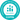# Exponent Rules: 7 Laws of Exponents to Solve Tough Equations

All Posts### Teachers

Category

• Teacher Resources
• Teaching Strategies

The exponent rules explain how to solve various equations that — as you might expect — have exponents in them. But there are several different kinds of exponent equations and exponential expressions, which can seem daunting... at first.

Mastering these basic exponent rules along with basic rules of logarithms (also known as “log rules”) will make your study of algebra very productive and enjoyable. Keep in mind that during this process, the order of operations will still apply.

Like most math tactics, there are teaching strategies you can use to make exponent rules easy to follow.

## What are exponents?

Exponents, also known as powers, are values that show how many times to multiply a base number by itself. For example, 43 is telling you to multiply four by itself three times.

43= 4 × 4 × 4 = 64

The number being raised by a power is known as the base, while the superscript number above it is the exponent or power.The equation above is said as “four to the power of three”. The power of two can also be said as “squared” and the power of three can be said as “cubed”. These terms are often used when finding the area or volume of various shapes.

Writing a number in exponential form refers to simplifying it to a base with a power. For example, turning 5 × 5 × 5 into exponential form looks like 53.

Exponents are a way to simplify equations to make them easier to read. This becomes especially important when you’re dealing with variables such as ‘𝒙’ and ‘𝑦’ — as 𝒙7× 𝑦5= ? is easier to read than (𝒙)(𝒙)(𝒙)(𝒙)(𝒙)(𝒙)(𝒙)(𝑦)(𝑦)(𝑦)(𝑦)(𝑦) = ?

## Rules of exponents in everyday life

Not only will understanding exponent properties help you to solve various algebraic problems, exponents are also used in a practical manner in everyday life when calculating square feet, square meters, and even cubic centimeters.

Exponent rules also simplify calculating extremely large or extremely tiny quantities. These are also used in the world of computers and technology when describing megabytes, gigabytes, and terabytes.

## What are the different rules of exponents?

There are seven exponent rules, or laws of exponents, that your students need to learn. Each rule shows how to solve different types of math equations and how to add, subtract, multiply and divide exponents.Make sure you go over each exponent rule thoroughly in class, as each one plays an important role in solving exponent based equations.

### 1. Product of powers rule

When multiplying two bases of the same value, keep the bases the same and then add the exponents together to get the solution.

42× 45 = ?

Since the base values are both four, keep them the same and then add the exponents (2 + 5) together.

42 × 45= 47

Then multiply four by itself seven times to get the answer.

47 = 4 × 4 × 4 × 4 × 4 × 4 × 4 = 16,384

Let’s expand the above equation to see how this rule works:In an equation like this, adding the exponents together is a shortcut to get the answer.

Here’s a more complicated question to try:

(4𝒙2)(2𝒙3) = ?

Multiply the coefficients together (four and two), as they are not the same base. Then keep the ‘𝒙’ the same and add the exponents.

(4𝒙2)(2𝒙3) = 8𝒙5

### 2. Quotient of powers rule

Multiplication and division are opposites of each other -- much the same, the quotient rule acts as the opposite of the product rule.

When dividing two bases of the same value, keep the base the same, and then subtract the exponent values.

55 ÷ 53 = ?

Both bases in this equation are five, which means they stay the same. Then, take the exponents and subtract the divisor from the dividend.

55÷ 53 = 52

Finally, simplify the equation if needed:

52= 5 × 5 = 25

Once again, expanding the equation shows us that this shortcut gives the correct answer:Take a look at this more complicated example:

5𝒙4 / 10𝒙2 = ?

The like variables in the denominator cancel out those in the numerator. You can show your students this by crossing out an equal number of 𝒙’s from the top and bottom of the fraction.

5𝒙4 / 10𝒙2 = 5𝒙/10

Then simplify where possible, as you would with any fraction. Five can go into ten, five times turning the fraction into ½ with the remaining 𝒙 variables.

5𝒙4/10𝒙2= 1𝒙2/2 = 𝒙2/2

### 3. Power of a power rule

This rule shows how to solve equations where a power is being raised by another power.

(𝒙3)3 = ?

In equations like the one above, multiply the exponents together and keep the base the same.

(𝒙3)3 = 𝒙9

Take a look at the expanded equation to see how this works:### 4. Power of a product rule

When any base is being multiplied by an exponent, distribute the exponent to each part of the base.

(𝒙𝑦)3 = ?

In this equation, the power of three needs to be distributed to both the 𝒙 and the 𝑦 variables.

(𝒙𝑦)3 = 𝒙3𝑦3

This rule applies if there are exponents attached to the base as well.

(𝒙2𝑦2)3 = 𝒙6𝑦6

Expanded, the equation would look like this:Both of the variables are squared in this equation and are being raised to the power of three. That means three is multiplied to the exponents in both variables turning them into variables that are raised to the power of six.

### 5. Power of a quotient rule

A quotient simply means that you’re dividing two quantities. In this rule, you’re raising a quotient by a power. Like the power of a product rule, the exponent needs to be distributed to all values within the brackets it’s attached to.

(𝒙/𝑦)4 = ?

Here, raise both variables within the brackets by the power of four.Take a look at this more complicated equation:

(4𝒙3/5𝑦4)2 = ?

Don’t forget to distribute the exponent you’re multiplying by to both the coefficient and the variable. Then simplify where possible.

(4𝒙3/5𝑦4)2= 42𝒙6/52𝑦8 = 16𝒙6/25𝑦8

### 6. Zero power rule

Any base raised to the power of zero is equal to one.The easiest way to explain this rule is by using the quotient of powers rule.

43/43 = ?

Following the quotient of powers rule, subtract the exponents from each other, which cancels them out, only leaving the base. Any number divided by itself is one.

43/43= 4/4 = 1

No matter how long the equation, anything raised to the power of zero becomes one.

(82𝒙4𝑦6)0 = ?

Typically, the outside exponent would have to be multiplied throughout each number and variable in the brackets. However, since this equation is being raised to the power of zero, these steps can be skipped and the answer simply becomes one.

(82𝒙4𝑦6)0 = 1

The equation fully expanded would look like this:

(82𝒙4𝑦6)0 = 80𝒙0𝑦0 = (1)(1)(1) = 1

### 7. Negative exponent rule

When there is a number being raised by a negative exponent, flip it into a reciprocal to turn the exponent into a positive. Don’t use the negative exponent to turn the base into a negative.We’ve talked about reciprocals before in our article, “How to divide fractions in 3 easy steps”. Essentially, reciprocals are what you multiply a number by to get the value of one. For example, to turn two into one, multiply it by ½.

Now, look at this exponent example:

𝒙-2 = ?

To make a number into a reciprocal:

1. Turn the number into a fraction (put it over one)
2. Flip the numerator into the denominator and vice versa
3. When a negative number switches places in a fraction it becomes a positive numberThe goal of equations with negative exponents is to make them positive.

Now, take a look at this more complicated equation:

4𝒙-3𝑦2/20𝒙𝑧-3 = ?

In this equation, there are two exponents with negative powers. Simplify what you can, then flip the negative exponents into their reciprocal form. In the solution, 𝒙-3 moves to the denominator, while 𝑧-3 moves to the numerator.

Since there is already an 𝒙 value in the denominator, 𝒙3 adds to that value.

4𝒙-3𝑦2/20𝒙z-3 = 𝑦2𝑧3/5𝒙4

With these seven rules in your students’ back pockets, they’ll be able to take on most exponent questions they come across!

## Exponent rules chartProdigy is a curriculum-aligned math game you can use to assign questions, track progress, and identify trouble spots in your students’ learning. And you can create teacher and student accounts for free!

With so many different exponent rules to follow and several students to track, it can be hard to see who needs help with what. Prodigy makes it easy to track progress, and create a unique gaming experience for each student based on their needs.Statistics are tracked live, as students play the game, and feedback is available instantly. Most of the time your students won’t even realize that they’re taking part in math lessons. It’s all part of their personalized gaming experience!

From the teacher dashboard, you can create lesson plans, see live statistics, input custom assignments, and prepare your students for upcoming tests. Here's how you can use Prodigy to:## Free exponent rules worksheet

Math worksheets are handy tools that can show how students are understanding key concepts. You can see how students are coming up with answers, where they’re struggling, and if any concepts need to be covered in more detail.

We’ve put together an exponent rules worksheet, with the help of our team of teachers, to help you with exponent lessons.

## Conclusion: exponent rules practice

Exponents are used to show how many times a base value is multiplied by itself. This simplifies equations to an easier to read format. (𝒙𝒙𝒙𝒙𝒙𝒙𝒙𝒙𝒙)(𝑦𝑦𝑦𝑦𝑦𝑦)(𝑧𝑧𝑧𝑧𝑧) = 𝒙9𝑦6𝑧5

To recap, there are seven basic rules that explain how to solve most math equations that involve exponents. The exponent rules are:

• Product of powers rule — Add powers together when multiplying like bases
• Quotient of powers rule — Subtract powers when dividing like bases
• Power of powers rule — Multiply powers together when raising a power by another exponent
• Power of a product rule — Distribute power to each base when raising several variables by a power
• Power of quotient rule — Distribute power to all values in a quotient
• Zero power rule — Any base raised to the power of zero becomes one
• Negative exponent rule — To change a negative exponent to a positive one, flip it into a reciprocal

Exponents have a tendency of appearing throughout our lives, so it’s important that students understand how they work moving forward. There are a lot of rules to remember but, once your students understand them, solving exponents will likely get easier!

#### Prodigy Math Game is an adaptive, game-based learning platform. Success in Prodigy requires students to correctly answer curriculum-aligned questions adapted to their learning needs, and gives teachers more ways to make math class fun! Sign up for your free teacher account today to get started.

•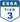•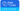•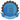•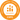•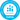•